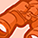# New to Qlik Sense

If you’re new to Qlik Sense, start with this Discussion Board and get up-to-speed quickly.

Announcements
Skip the ticket, Chat with Qlik Support instead for instant assistance.
cancel
Showing results for
Did you mean:Contributor III

## Use calculated value into new calculation

To create a forecasted revenue I have the following calculation.

num(SUM({1<MonthNum ={"<=\$(=Num(Month(Today())))"}, Year={"\$(=Year(Today()))"}>}[Revenue])

/ SUM({1<MonthNum ={"<=\$(=Num(Month(Today())))"}, Year={"\$(=Year(Today())-1)"}>}[Revenue])-1,'#,##0.0%')

This gives a ratio of this year YTD revenue vs last year YTD revenue. Now I want to use that ratio into a calculation about 2016.

Say [actual revenue] *

num(SUM({1<MonthNum ={"<=\$(=Num(Month(Today())))"}, Year={"\$(=Year(Today()))"}>}[Revenue])

/ SUM({1<MonthNum ={"<=\$(=Num(Month(Today())))"}, Year={"\$(=Year(Today())-1)"}>}[Revenue])-1,'#,##0.0%')

However, if I plot this in a table with a year dimension I get nothing.

1 Solution

Accepted SolutionsMVP

May be try this:

[actual revenue] *

If(Year = 2016, Num(Sum(TOTAL {1<MonthNum ={"<=\$(=Num(Month(Today())))"}, Year={"\$(=Year(Today()))"}>}[Revenue])/Sum(TOTAL {1<MonthNum ={"<=\$(=Num(Month(Today())))"}, Year={"\$(=Year(Today())-1)"}>}[Revenue])-1, 1),'#,##0.0%')

I have used a static condition of Year = 2016, but you need to determine a more advanced condition for what determines a forecast here?

3 RepliesMVP

May be try this:

[actual revenue] *

If(Year = 2016, Num(Sum(TOTAL {1<MonthNum ={"<=\$(=Num(Month(Today())))"}, Year={"\$(=Year(Today()))"}>}[Revenue])/Sum(TOTAL {1<MonthNum ={"<=\$(=Num(Month(Today())))"}, Year={"\$(=Year(Today())-1)"}>}[Revenue])-1, 1),'#,##0.0%')

I have used a static condition of Year = 2016, but you need to determine a more advanced condition for what determines a forecast here?Contributor III
Author

Thanks! The Total was the key here!MVP

Indeed it wasTags
Community Browser